×

Search anything:

# Introduction to Perl Programming

#### Perl Programming Software EngineeringGet this book -> Problems on Array: For Interviews and Competitive Programming

Perl is a general-purpose programming language, it is used in text processing and manipulation, systems administration, network programming, GUI development, web development just to name a few. In this article, we go over a brief introduction to Perl.

1. Introduction.
2. How to run Perl code.
3. Syntax.
4. Variables.
5. Variable scoping.
6. Loops and conditional statements.
7. Built-in operators.
8. Summary.
9. References.

## Introduction.

Perl is a general-purpose programing language that finds applications in various areas. We look at how to run Perl in Linux, the basic Perl syntax, variables and variable scoping, and operators used with Perl.

We can check for the Perl version currently installed by writing:

``````\$ perl -v
``````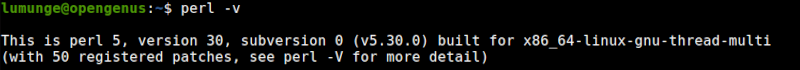However, if Perl is not installed, we can install as shown in article.

## How to run a Perl program.

On the Linux command-line, we can execute a Perl program as follows:

``````\$ perl -e "print 'hello world';"
``````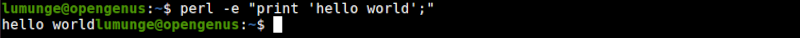We can also create a file with the .pl file extension.

``````\$ sudo vim perl_intro.pl
``````

``````#!/usr/bin/perl
use strict;
use warnings;

# COMMENT
print "hello world";
``````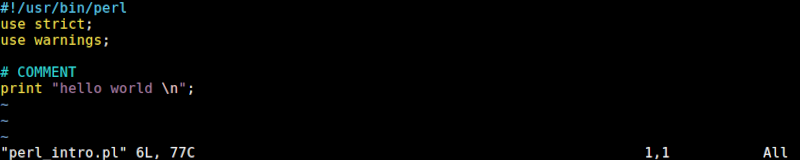To execute the script we write:

``````\$ perl perl_intro.pl
``````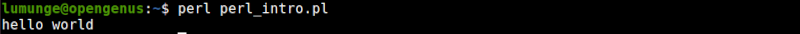## Syntax:

Here we look at the basic syntax, for more detailed documentation the link is helpful.
In Perl, comments are preceded with a # character as can be seen from the above code snippet.

#### Quotes.

For string literals we can use either single or double quotes, however when we are using special characters such as tabs(\t) or newline characters(\n) we use double quotes:
An example:

``````print "hello world \n"
``````

Numbers don't need quotes:

``````print 12
``````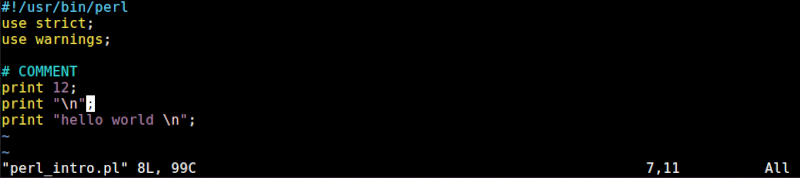## Variables.

In Perl we have scalars, arrays and hashes variables. Here we look at all three by examples, for more detailed documentation.

Scalars
An example of a scalar is:

``````my \$num = 88;
my \$str = "This is a string";
my \$floating = 88.45;
``````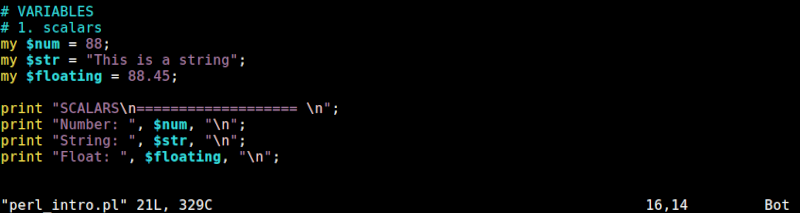Arrays.
We initialize arrays as follows:

``````my @strings = ("string1", "string2", "string3");
my @numbers = (34, 67, 98);
my @strnum = (34, "string1", 45);

if(@numbers > 4){
print @numbers
}
``````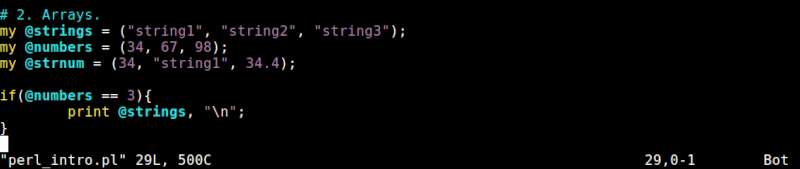We can also sort arrays and reverse as follows:

``````my @arr = (23, 11, 13, 47);
my @srtArr = sort @arr;
my @revArr = reverse @arr;
``````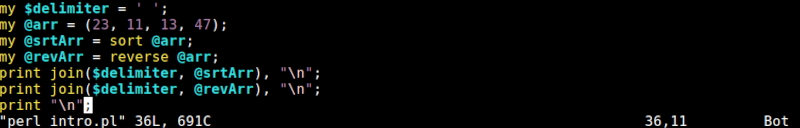Hashes
A hash is a key-value pair, for example:

``````my %user_profile = (
firstname => "bugs",
lastname => "bunny",
year => 2006,
flag => "false",
);
# get the value of year
print %user_profile{"year"}, "\n";

# get all keys
print keys %user_profile,"\n";

# get all values
print values %user_profile, "\n";
print "\n";
``````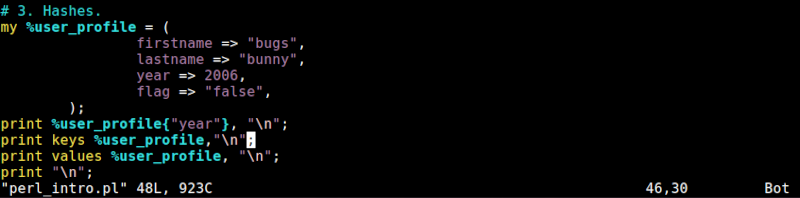Hashes can also be nested, for example:

``````my \$inventory = {
Java => {
description: "object oriented",
category => 'cat1',
},
C++ => {
description: "logic oriented",
category => "cat2",
},
description: "functional",
category => "cat3",
},
};

``````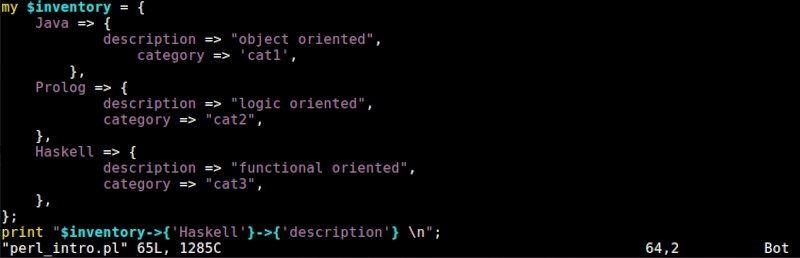## Scoping.

Instead of using the my keyword we can just declare variables without is, for example:

``````\$name  = "Alice";
``````

Here, we have initialized the variable name without using the my keyword, this makes it a global variable. As the size of the program grows so as the number of global variables and this is considered bad programming practice.

We use the my keyword to lexically scope a variable and thus it will only be available to its current block.
An example:

``````my \$year = 2010;
my \$thisYear = 2022;
if(\$thisYear - \$year > 10){
my \$res = "true";
print \$year;
print \$res;
}
# out of scope
print \$res;
``````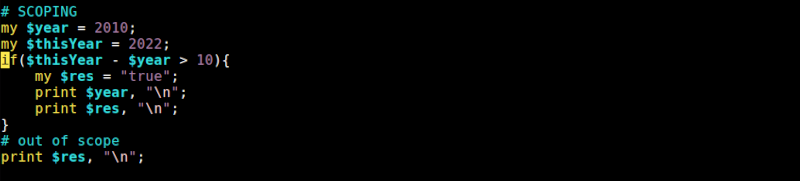We will have the following error.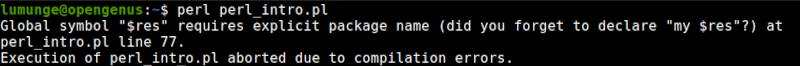## Loops and conditional statements.

while loops.
A while loop executes as long as the specified condition holds. In Perl we write while loops as follows:

``````while(condition){
// action;
}
``````

until loops.
Just like shell we also have until loops in Perl:

``````until(condition){
/// action;
}
``````

for loops
For loops are written in a similar way we write loops in Java or C/C++ programming languages:

``````for(\$i = 0; \$i = n; \$i++){
// actions;
}
``````

foreach loops.
For each loop in Perl is written as follows:

``````foreach(@array){
print "array element";
}
``````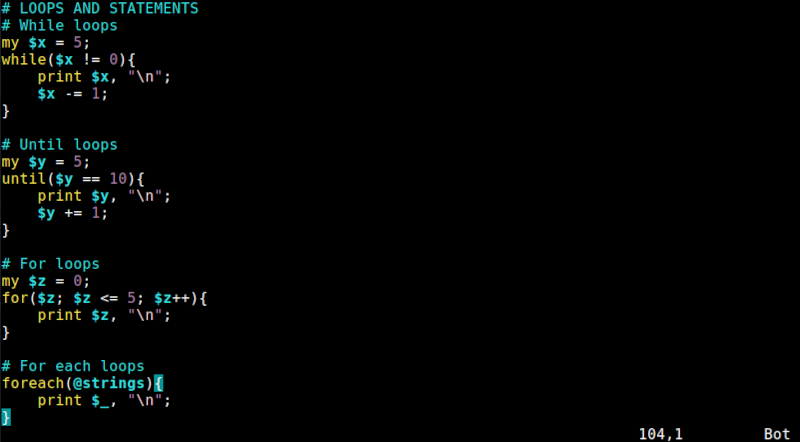if-else, unless statements.
In Perl we have if-else statements which we write as follows:

``````if(condition){
// action;
}elseif(condition1){
// action1;
}else{
// action2;
}
``````

We also have the unless statement that negates as follows:

``````unless(condition){
// action;
}
``````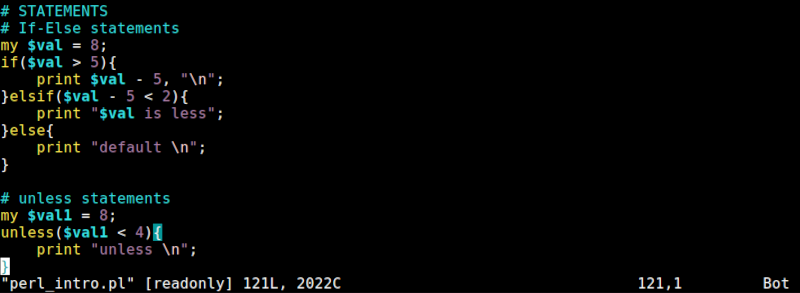We can also write if(!condition) as a negation.

## Built-in operators.

In Perl we have the following operators:

• - - subtraction.
• * - multiplication.
• / - division.

Numeric comparison operators are:

• == equality.
• != - inequality.
• < - less than.
• > - greater than.
• <= - less than or equal.
• >= - greater than or equal.

String comparison operators are:

• eq - equality.
• ne - inequality.
• lt - less than.
• gt - greater than.
• le - less than or equal.
• ge - greater than or equal.

Boolean operators are:

• && - and.
• || - or.
• ! - not.

Other operators are:

• = - assignment.
• . - concatenate strings.
• x - multiply strings(repeat strings).
• .. - range operator.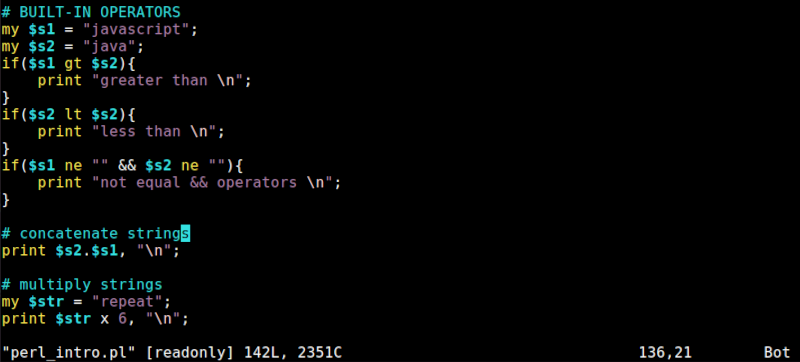## Summary.

Perl is used in text processing and manipulation, systems administration, network programming, GUI development, web development just to name a few. We have looked at the basics of Perl language

docs

#### Erick Lumunge

Erick is a passionate programmer with a computer science background who loves to learn about and use code to impact lives positively.